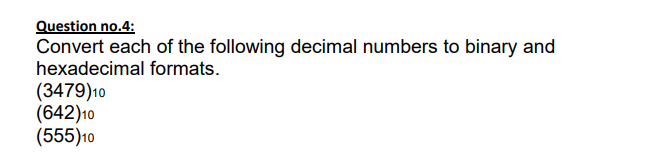# Question Question no.4: Convert each of the following decimal numbers to binary and hexadecimal formats. $$(3479)_{10}$$ $$(642) 10$$ $$(555)_{10}$$NZ5DZI The Asker · Computer ScienceTranscribed Image Text: Question no.4: Convert each of the following decimal numbers to binary and hexadecimal formats. $$(3479)_{10}$$ $$(642) 10$$ $$(555)_{10}$$
More
Transcribed Image Text: Question no.4: Convert each of the following decimal numbers to binary and hexadecimal formats. $$(3479)_{10}$$ $$(642) 10$$ $$(555)_{10}$$